# Texas Go Math Grade 2 Lesson 20.1 Answer Key Saving and Spending

Refer to our Texas Go Math Grade 2 Answer Key Pdf to score good marks in the exams. Test yourself by practicing the problems from Texas Go Math Grade 2 Lesson 20.1 Answer Key Saving and Spending.

## Texas Go Math Grade 2 Lesson 20.1 Answer Key Saving and Spending

Essential Question
How are saving and spending different?
Explanation:
Savings is the amount of money left over after spending and other obligations are deducted from earnings. Savings represent money that is otherwise idle and not being put at risk with investments or spent on consumption.
Spending money is money that you have or are given to spend on personal things for pleasure

Explore
Find the total value of each group of coins.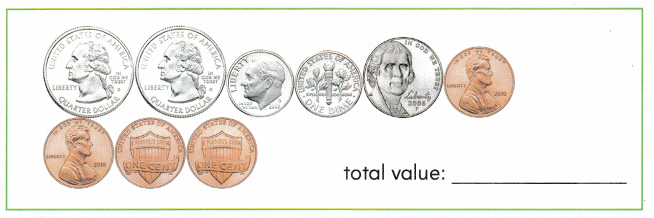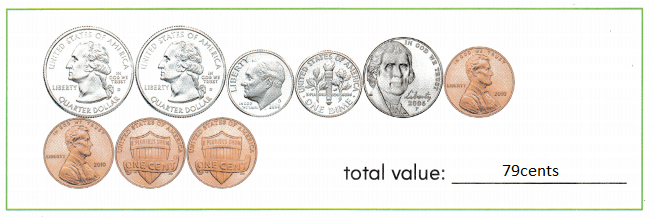Explanation:
1 quarter dollar = 25¢
2 x 25 = 50¢
1 dime = 10¢
2 x 10 = 20¢
1 Nickel= 5¢
1 penny = 1¢
4 x 1 = 4¢
50 + 20 + 5 + 4 = 79¢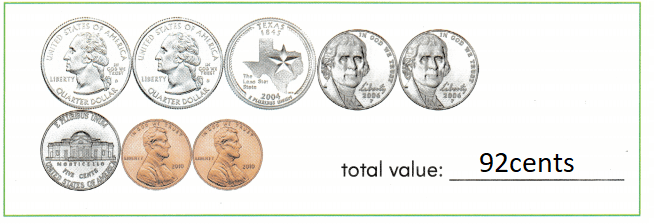Explanation:
3 quarter dollar = 25¢
3 x 25 = 75¢
1 Nickel= 5¢
3 x 5 = 15
1 penny = 1¢
2 x 1 = 2¢
75 + 15 + 2 = 92¢

FOR THE TEACHER

• Review the names and values of the different coin types with children. Then have children find the total value for each group of coins.

Math Talk
Mathematical Processes
Describe how to compare the total values of these of coins.
The values of the coins are compared by subtracting
Explanation:
first table shows = 79
second table shows = 92
92 – 79 = 13¢

Model and Draw

Suppose you get one dime each Friday.

You can save the dime each week.In 4 weeks, you will have ___.
Explanation:
1 dime = 10¢
In 4 weeks, you will have
4 x 10 = 40¢

You can spend the dime each week to buy things.In 4 weeks, you will have ___.
Explanation:
1 dime = 10¢
In 4 weeks, you will spend
4 x 10 = 40¢
40 – 40 = 0

Share and Show

Use the coins to answer the question.

Question 1.
Nick gets one dime each week for 6 weeks. He saves 4 dimes and spends 2 dimes. How much money does he have now?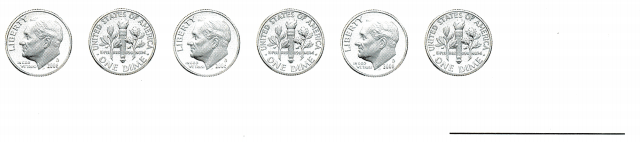Explanation:
6 x 1 = 6
He saves 4 dimes and spends 2 dimes.
6 – 2 = 4
4 dimes he saves

Question 2.
Cindy gets one dime each week for 7 weeks. She saves 3 dimes and spends 4 dimes. How much money does she have now?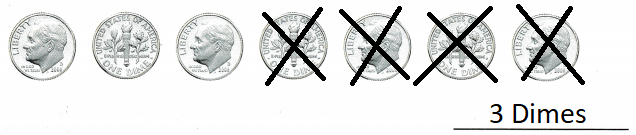Explanation:
one dime each week for 7 weeks.
saves 3 dimes and spends 4 dimes.
7 – 4 = 3
she have now 3 dimes

Problem Solving

Use the coins to answer the question.

Question 3.
Kim gets one quarter each week for 4 weeks. She saves 3 quarters and spends 1 quarter. How much money does she have now?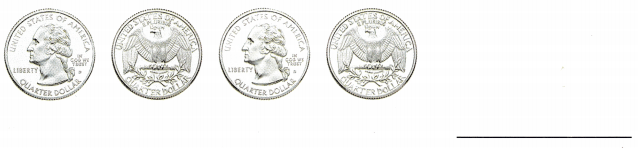Explanation:
one quarter each week for 4 weeks.
She saves 3 quarters and spends 1 quarter.
4 – 1 = 3
she saves 3 quarters

Draw coins to solve.

Question 4.
H.O.T. Multi-Step Theo gets one dime and one nickel each week For 4 weeks. He spends 15¢ and saves the rest. How much money does he have now?
45¢  Theo have
Explanation:
one dime and one nickel each week For 4 weeks.
1 dime = 10¢
1 nickel = 5¢
10 + 5 = 15¢
4 x 15 = 60¢
60 – 15 = 45¢ money that he have now

Question 5.
H.O.T. Multi-Step Kendra gets 4 nickels each week for 3 weeks. She saves half of this money and spends the rest. How much money does she spend?
30¢ she spend
Explanation:
Kendra gets 4 nickels each week for 3 weeks.
4 x 3 = 12
1 nickel = 5
12 x 5 = 60
60 ÷ 2 = 30
30¢ she spend

Choose the correct answer.

Question 6.
Multi-Step Larry earns 50e each week. He wants to buy a book that costs 75e. After two weeks he buys the book and saves the rest. How much does he save?
(A) 50¢
(B) 25¢
(C) 45¢